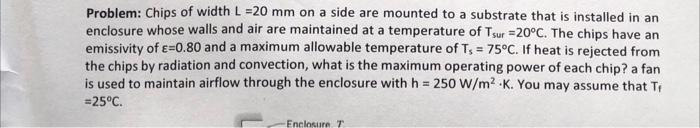Home / Expert Answers / Mechanical Engineering / problem-chips-of-width-mathrm-l-20-mathrm-mm-on-a-side-are-mounted-to-a-substrate-that-pa491

# (Solved): Problem: Chips of width $$\mathrm{L}=20 \mathrm{~mm}$$ on a side are mounted to a substrate that ...Problem: Chips of width $$\mathrm{L}=20 \mathrm{~mm}$$ on a side are mounted to a substrate that is installed in an enclosure whose walls and air are maintained at a temperature of $$T_{\text {sur }}=20^{\circ} \mathrm{C}$$. The chips have an emissivity of $$\varepsilon=0.80$$ and a maximum allowable temperature of $$T_{5}=75^{\circ} \mathrm{C}$$. If heat is rejected from the chips by radiation and convection, what is the maximum operating power of each chip? a fan is used to maintain airflow through the enclosure with $$\mathrm{h}=250 \mathrm{~W} / \mathrm{m}^{2} \cdot \mathrm{K}$$. You may assume that $$\mathrm{T}_{\mathrm{f}}$$ $$=25^{\circ} \mathrm{C} .$$

We have an Answer from Expert